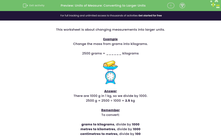# Convert to Larger Units of Measurement

In this worksheet, students will change the given measurements into larger units.Key stage:  KS 2

Curriculum topic:   Measurement

Curriculum subtopic:   Convert Units of Measure

Difficulty level:#### Worksheet Overview

This activity is about changing measurements into larger units.

Example

Change the mass from grams into kilograms.

2,500 grams = ______ kilogramsThere are 1,000 g in 1 kg, so we divide by 1,000.

2,500 g = 2,500 ÷ 1,000 = 2.5 kg

Remember

To convert:

Grams to kilograms, divide by 1,000

Metres to kilometres, divide by 1,000

Centimetres to metres, divide by 100  (watch out for this one - we divide by 100, not 1,000!)

Are you ready to give this a go?### What is EdPlace?

We're your National Curriculum aligned online education content provider helping each child succeed in English, maths and science from year 1 to GCSE. With an EdPlace account you’ll be able to track and measure progress, helping each child achieve their best. We build confidence and attainment by personalising each child’s learning at a level that suits them.

Get started